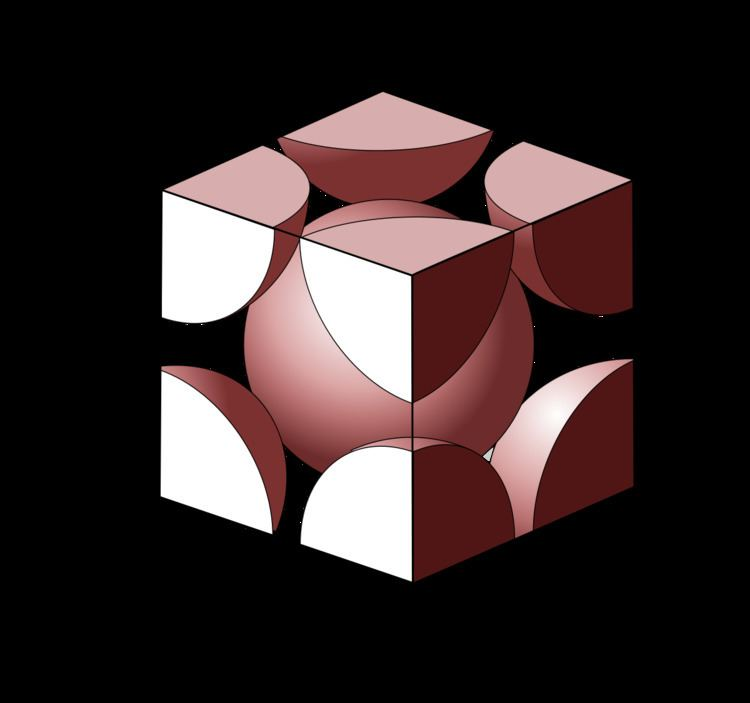# Atomic packing factor

Updated onIn crystallography, atomic packing factor (APF), packing efficiency or packing fraction is the fraction of volume in a crystal structure that is occupied by constituent particles. It is a dimensionless quantity and always less than unity. In atomic systems, by convention, the APF is determined by assuming that atoms are rigid spheres. The radius of the spheres is taken to be the maximal value such that the atoms do not overlap. For one-component crystals (those that contain only one type of particle), the packing fraction is represented mathematically by

## Contents

A P F = N p a r t i c l e V p a r t i c l e V u n i t   c e l l

where Nparticle is the number of particles in the unit cell, Vparticle is the volume of each particle, and Vunit cell is the volume occupied by the unit cell. It can be proven mathematically that for one-component structures, the most dense arrangement of atoms has an APF of about 0.74 (see Kepler conjecture), obtained by the close-packed structures. For multiple-component structures, the APF can exceed 0.74.

## Single component crystal structures

Common sphere packings taken on by atomic systems are listed below with their corresponding packing fraction.

• Hexagonal close-packed (hcp): 0.74
• Face-centered cubic (fcc): 0.74 (also called cubic close-packed, ccp)
• Body-centered cubic (bcc): 0.68
• Simple cubic: 0.52
• Diamond cubic: 0.34
• The majority of metals take on either the hcp, fcc, or bcc structure.

## Body-centered cubic

The primitive unit cell for the body-centered cubic crystal structure contains several fractions taken from nine atoms: one on each corner of the cube and one atom in the center. Because the volume of each of the eight corner atoms is shared between eight adjacent cells, each BCC cell contains the equivalent volume of two atoms (one central and one on the corner).

Each corner atom touches the center atom. A line that is drawn from one corner of the cube through the center and to the other corner passes through 4r, where r is the radius of an atom. By geometry, the length of the diagonal is a3. Therefore, the length of each side of the BCC structure can be related to the radius of the atom by

a = 4 r 3 .

Knowing this and the formula for the volume of a sphere, it becomes possible to calculate the APF as follows:

A P F = N a t o m s V a t o m V c r y s t a l = 2 4 3 π r 3 ( 4 r 3 ) 3 = π 3 8 0.680 174 76 .

## Hexagonal close-packed

For the hexagonal close-packed structure the derivation is similar. Here the unit cell (equivalent to 3 primitive unit cells) is a hexagonal prism containing six atoms. Let a be the side length of its base and c be its height. The volume of the unit cell of hcp can be taken as 242r3. Then:

a = 2 r , c = 4 2 3 r .

It is then possible to calculate the APF as follows:

A P F = N a t o m s V a t o m V c r y s t a l = 6 4 3 π r 3 3 3 2 a 2 c = 6 4 3 π r 3 3 3 2 ( 2 r ) 2 2 3 4 r = 6 4 3 π r 3 3 3 2 2 3 16 r 3 = π 18 = π 3 2 0.740 480 48 .

## References

Similar Topics
Starlift
Malcolm Blight
Patrick McGrath (ice hockey)
Topics